# NEET Important Chapter - Moving Charges and Magnetism

Get plus subscription and access unlimited live and recorded courses## Moving Charges and Magnetism for NEET

The chapter moving charges and magnetism deals with the magnetic field produced by the current-carrying wire and the  magnetic force acting on a current-carrying wire and moving charge in a magnetic field. As far as the NEET exam is concerned, problems will be asked related to concepts discussed in the chapter.

The chapter moving charges and magnetism begins with the magnetic force acting on a charged particle moving in a magnetic field. Cyclotron which is a practical application of lorentz force is discussed in this chapter in detail. Biot-Savart law and Ampere’s law are very important concepts in this chapter.

We also learn about the formula of magnetic effect of electric current and magnetic field produced due to a solenoid and toroid. Force acting on a current-carrying conductor placed in a  magnetic field and the force acting between two parallel straight conductors are also discussed in this chapter.

Now, let us move on to the important concepts and moving charges and magnetism  formulas related to the NEET 2022 exam from the chapter moving charge and magnetism along with a few solved examples.

### Important Topics of Moving Charges and Magnetism

• Magnetic force on a moving charge in a magnetic field

• Trajectory of a charge moving in a perpendicular magnetic field

• Cyclotron

• Biot Savart Law

• Ampere’s Law

• Magnetic field due to a circular and straight wire

• Magnetic field due to a solenoid

• Force on a current-carrying conductor in a magnetic field.

• Force between two parallel current-carrying conductors

### Important Concepts of Moving Charges and Magnetism

 Name of the Concept Key Points of the Concept 1. Magnetic force on a moving charge in a magnetic field A moving charge produces an electric field and magnetic field.The charge (q) moving with a velocity (v) in a magnetic field (B) experiences a magnetic force(F) given by $\vec F=q(\vec v\times \vec B)$$F=qvB\sin\theta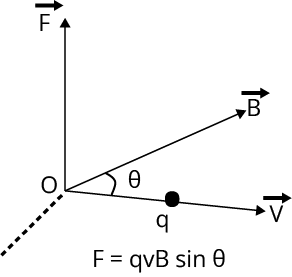The direction of magnetic force is given by right-hand rule as shown below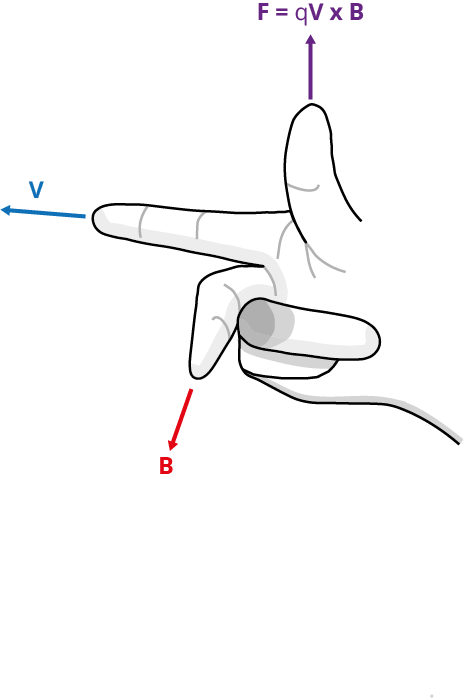2. The trajectory of a charge moving in a perpendicular magnetic field When a charge (q) moves in a perpendicular magnetic field (B), then it follows a circular path and necessary centripetal force is given magnetic force.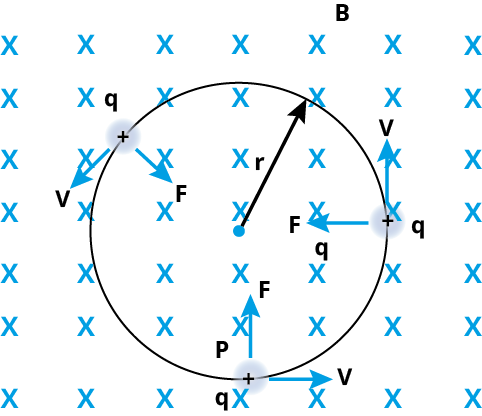The radius (r) of the circular path is given byr=\dfrac{mv}{qB}Time period of motion of charge in a circular path isT=\dfrac{2\pi m}{qB}Kinetic energy of circular path is given byK=\dfrac{q^2B^2R^2}{2m} 3. Cyclotron Cyclotron is a device used to accelerate positively charged particles.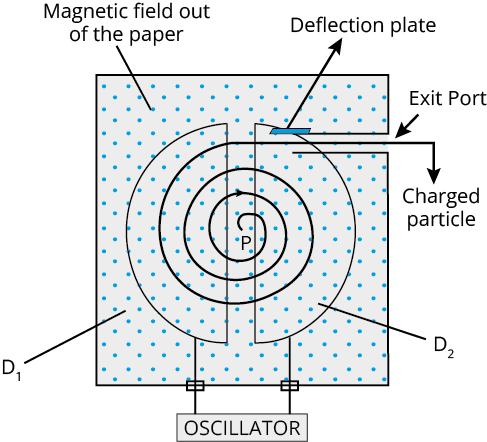The charged particles are accelerated by an alternating electric field created by two D shaped semicircular hollow chambers in a perpendicular magnetic field.Since the charged particle is moving in a perpendicular magnetic field, it follows a circular path of increasing radiusThe cyclotron frequency is given byf=\dfrac{qB}{2\pi m} 4. Biot Savart Law According to Biot Savart law, the magnetic field (dB) at a point due to the current element (dl) is given by the expression\vec dB=\dfrac{\mu_0}{4\pi}\dfrac{\vec {Idl}\times\vec r}{r^3}$$dB=\dfrac{\mu_0}{4\pi}\dfrac{Idl\sin\theta}{r^2}$Where μ is the magnetic permeability of the medium and for free space, magnetic permeability μ0 = 4ℼ✕ 10-7 H/mIf you hold a current-carrying conductor in such a way that your thumb points towards the direction of current, then the remaining fingers give the direction of the magnetic field at that point.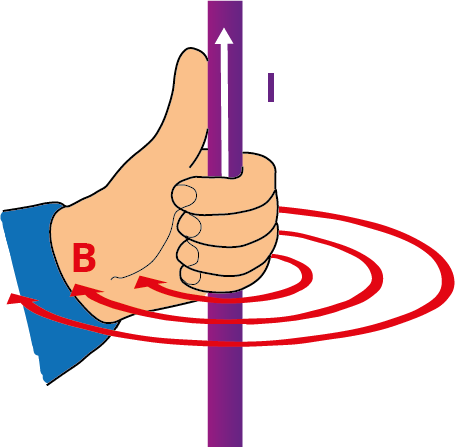In the case of a circular current-carrying coil, if the four folding fingers of the right-hand point towards the direction of the current, then the thumb point towards the direction of magnetic field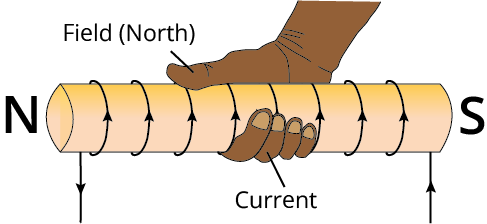5. Ampere’s Law Ampere’s law gives a method to calculate the magnetic field due to a given current distribution.According to ampere’s law, the line integral of the magnetic field around any closed curve is equal to μ0 times the net current enclosed by the closed curve.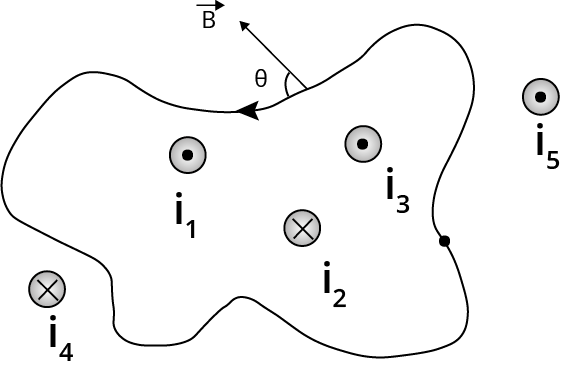$\oint\vec B\cdot\vec{dl}=\mu_0i_{enclosed}$$\oint\vec B\cdot\vec{dl}=\mu_0(i_1+i_3-i_2) 6. Magnetic field due to a circular and straight wire Magnetic field due to a circular coil having current I and radius R at a distance x along its axis is given byB=\dfrac{\mu_0IR^2}{2(R^2+x^2)^{3/2}}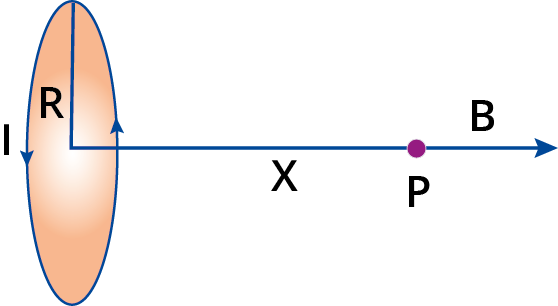Magnetic field due to an infinitely long current-carrying wire having current I at a distance d isB=\dfrac{\mu_0I}{2\pi d}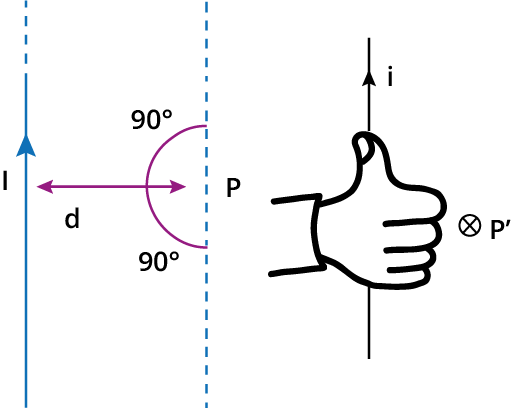7. Magnetic field due to a solenoid A solenoid is a tightly wounded cylindrical coil of insulated wire having a smaller diameter than its lengthMagnetic field due to a solenoid having n number of turns per unit length along its axis outside the solenoid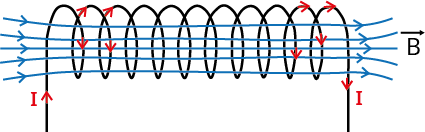B=\mu_0nI 8. Force on a current-carrying conductor in a magnetic field. When a current-carrying conductor having length L is placed in a magnetic field B, it will experience a force given by the formula,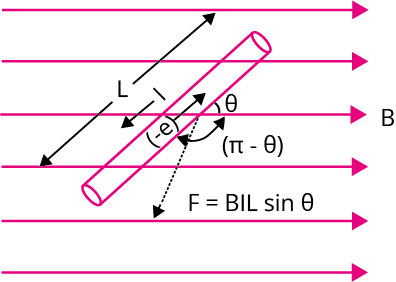F=ILB\sin\thetaThe direction of force is given by fleming’s left-hand rule as given below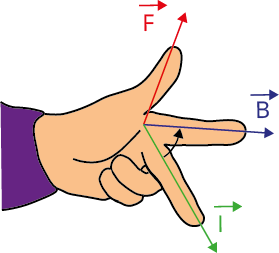9. Force between two parallel current-carrying conductors Force acting between two parallel current-carrying conductors having length l is given byF_1=\dfrac{\mu_0}{4\pi}\dfrac{2I_1I_2}{d}\times l$$F_2=\dfrac{\mu_0}{4\pi}\dfrac{2I_1I_2}{d}\times l$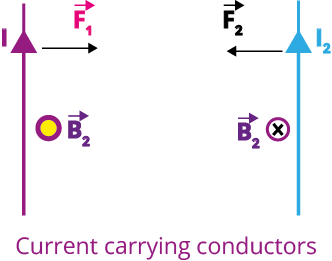If the current in the conductors is in the same direction, there will be the force of attraction and if the currents are in the opposite direction, then there will be the force of repulsion

### List of Important Formulae

 Sl. No Name of the Concept Formula Lorentz force formula The force acting on a charged particle (q) in a combined electric field (E) and magnetic field (B)$\vec F=q\vec E+q(\vec v\times \vec B)$ Pitch and radius of a helical path of a charged particle (q) moving at an angle θ to the magnetic field(B) with a velocity (v) $\text{Pitch}=\dfrac{2\pi mv\cos\theta}{qB}$$r=\dfrac{mv\sin\theta}{qB}$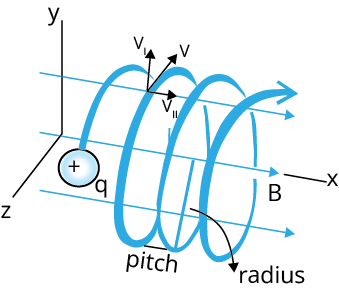Magnetic field inside a torroid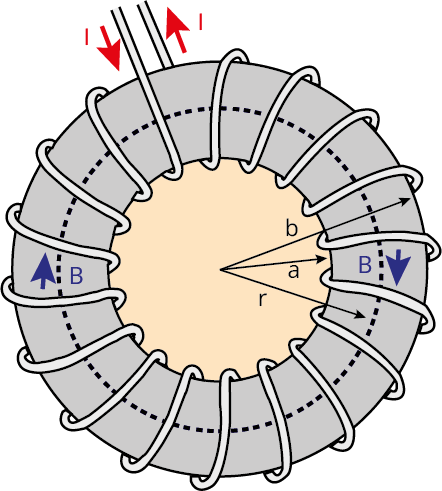$B=\mu_0nI$ The magnetic moment of a current-carrying coil having an area of cross-section (A) and N number of turns $M=NAI$ The torque acting on a current-carrying coil placed in a magnetic field B $\tau = NAIB\sin\theta$Where θ is the angle between area vector and magnetic field and N is the number of turns The magnetic field at a point which lies on the perpendicular bisector of the finite length of straight wire $B=\dfrac{\mu_0I}{4\pi R}(\sin\phi_1+\sin\phi_2)$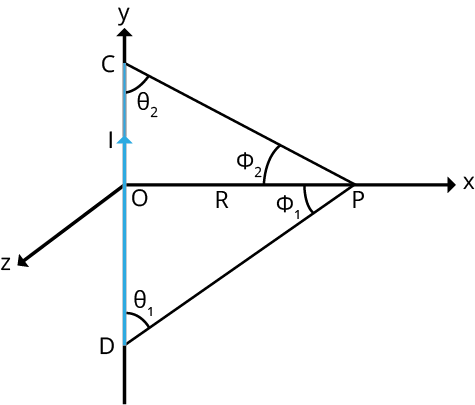### Solved Examples

1. Two long parallel wires carrying equal current separated by 1 m, exert a force of 2✕10-7 N/m on one another. The current flowing through them is

Sol:

Let the Current passing through each wire be I

The force per unit length acting on each wire is given as 2✕10-7 N/m

The formula to calculate the force exerted by wires on one another is given by,

$F=\dfrac{\mu_0}{4\pi}\dfrac{2I^2}{d}\times l$

$\dfrac{F}{l}=\dfrac{\mu_0}{4\pi}\dfrac{2I^2}{d}$

$2\times10^{-7}=\dfrac{2\times10^{-7}\times I^2}{1}$

$I=1\text{ A}$

Therefore, the total current flowing through them is 1 A.

Key Point: Force acting between two parallel current-carrying wires depend on the current passing through the wries and distance between them.

2. An alpha particle and proton travel with same velocity in a magnetic field perpendicular to the direction of their velocities, find the ratio of the radii of their circular path.

Sol:

Let the mass of the proton and alpha particle be ‘m’ and ‘4m’ respectively. The charge of the alpha particle is two times the charge of the proton.

The formula to calculate the radius of the circular path of proton is given by

$r=\dfrac{mv}{qB}$...(1)

The radius of the circular path of the alpha particle is given by,

$r'=\dfrac{(4m)v}{(2q)B}$

$r'=\dfrac{2mv}{qB}$....(2)

Divide equation (1) by equation (2) to obtain the ratio of radii of their circular path.

$\dfrac{r}{r'}=\dfrac{\left(\dfrac{mv}{qB}\right)}{\left(\dfrac{2mv}{qB}\right)}$

$\dfrac{r}{r'}=\dfrac{1}{2}$

Therefore, the ratio of the radii of thor circular path is 1:2

Key Point: Alpha particle is a He2+ nucleus and has mass four times the mass of a proton and charge equal to double the charge of proton

### Previous Year Questions from NEET Paper

1. A 250 turn rectangular coil of length 2.1 cm and width 1.25 cm carries a current of 85 μA and subjected to magnetic field of strength 0.85 T. Work done for rotating the coil by 180o against the torque is (NEET 2017)

1. 4.55 μJ

2. 2.3 μJ

3. 1.15 μJ

4. 9.1 μJ

Sol:

The magnetic moment of the rectangular coil is calculated by the formula given below,

$M=NAI$

$M=250\times(2.1\times 10^{-2}\times1.25\times 10^{-2})\times85\times 10^{-6}$

$M= 5.58\times 10^{-6}$

Work done in rotating the rectangular coil can be calculated as follows,

$W = MB(\cos\theta_1-\cos\theta_2)$

$W = MB(\cos0^o-\cos180^o)$

$\tau = 5.58\times 10^{-6}\times 0.85 \times(1+1)$

$W=9.1~\mu J$

Therefore, correct option is option (d)

Trick: Magnetic moment of rectangular coil is equal to the product of number of turns, area of the coil and the current passing through the coil.

2. An infinitely long straight conductor carries a current of 5 A as shown. An electron is moving with a speed of 105 m/s parallel to the conductor. The perpendicular distance between the electron and the conductor is 20 cm at an instant. Calculate the magnitude of the force experienced by the electron at that instant. (NEET 2021)

1. 4✕10-20 N

2. 8𝜋✕10-20 N

3. 4𝜋✕10-20 N

4. 8✕10-20 N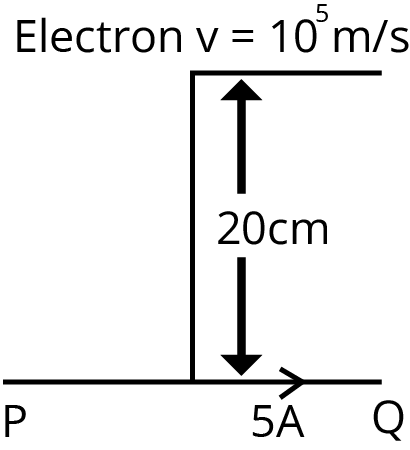Sol:

The magnetic field due to current carrying infinitely long straight conductor is given by,

$B=\dfrac{\mu_0I}{2\pi d}$

$B=\dfrac{4\pi\times 10^{-7}\times 5}{2\pi \times20\times10^{-2}}$

$B=5\times 10^{-6}~\text{T}$

The force acting on the electron is given by

$F=qvB$

$F=1.6\times 10^{-19}\times 10^{6}\times5\times 10^{-6}$

$B=8\times10^{-20}~N$

The correct answer is option (d).

Trick: The motion of a charged particle and the magnetic field produced by the infinitely long conducting wire is perpendicular to each other.

### Practice Questions

1. A closely wound flat circular coil of 25 turns of wire has diamter of 10 cm which carries a current of 4 A. The flux density at the centre of the coil will be ? (Ans: 1.256✕10-3 T)

2. An electron is moving in a circular orbit of radius r makes n rotations per second. The magnetic field produced at the centre has magnitude_____ (Ans: μ0ne/2r)

### Conclusion

In this article we have provided important information regarding the chapter moving charges and magnetism such as important concepts, formulae, etc.. Students should work on more solved examples along with  previous year question papers for scoring good grades in the NEET exam.

See More## NEET Important Dates

View All Dates
NEET 2022 exam date and revised schedule have been announced by the NTA. NEET 2022 will now be conducted on 17-July-2022, and the exam registration closes on 20-May-2022. You can check the complete schedule on our site. Furthermore, you can check NEET 2022 dates for application, admit card, exam, answer key, result, counselling, etc along with other relevant information.
See More
View All Dates## NEET Information

Application Form
Eligibility Criteria
Reservation Policy
NTA has announced the NEET 2022 application form release date on the official website https://neet.nta.nic.in/. NEET 2022 Application Form is available on the official website for online registration. Besides NEET 2022 application form release date, learn about the application process, steps to fill the form, how to submit, exam date sheet etc online. Check our website for more details.## NEET 2022 Study Material

View NEET Syllabus in Detail
View NEET Syllabus in Detail## NEET 2022 Study Material

View all study material for NEET
All
Physics
Chemistry
Biology
See All## NEET Question Papers## NEET 2022 Book Solutions and PDF Download

View all NEET Important Books
Biology
NCERT Book for Class 12 Biology
Physics
NCERT Book for Class 12 Physics
Chemistry
NCERT Book for Class 12 Chemistry
Physics
H. C. Verma Solutions
See All## NEET Mock Tests

View all mock tests
"NEET 2022 free online mock test series for exam preparation are available on the Vedantu website for free download. Practising these mock test papers of Physics, Chemistry and Biology prepared by expert teachers at Vedantu will help you to boost your confidence to face the NEET 2022 examination without any worries. The NEET test series for Physics, Chemistry and Biology that is based on the latest syllabus of NEET and also the Previous Year Question Papers."
See More## NEET 2022 Cut Off

NEET Cut Off
NTA is responsible for the release of the NEET 2022 cut off score. The qualifying percentile score might remain the same for different categories. According to the latest trends, the expected cut off mark for NEET 2022 is 50% for general category candidates, 45% for physically challenged candidates, and 40% for candidates from reserved categories. For the general category, NEET qualifying marks for 2021 ranged from 720-138 general category, while for OBC/SC/ST categories, they ranged from 137-108 for OBC, 137-108 for SC and 137-108 for ST category.
See More## NEET 2022 Results

The NEET 2022 result is published by NTA on https://neet.nta.nic.in/ in the form of a scorecard on 7-Sep-2022. The scorecard will include the roll number, application number, candidate's personal details, and the percentile, marks, and rank of the candidate. Only those candidates who achieve the NEET cut-off will be considered qualified for the exam.
See More
Rank List
Counselling
Cutoff
NEET 2022 state rank lists will be released by the state counselling committees for admissions to the 85% state quota and to all seats in private medical and dental colleges. NEET 2022 state rank lists are based on the marks obtained in entrance exams. Candidates can check the NEET 2022 state rank list on the official website or on our site.## NEET Top Colleges

View all NEET 2022 Top Colleges
Want to know which Engineering colleges in India accept the NEET 2022 scores for admission to Engineering? Find the list of Engineering colleges accepting NEET scores in India, compiled by Vedantu. There are 1622 Colleges that are accepting NEET. Also find more details on Fees, Ranking, Admission, and Placement.
See More## FAQs on NEET Important Chapter - Moving Charges and Magnetism

FAQ

1. How many questions are asked from magnetism in NEET?

Nearly 1-2 questions are asked in the NEET exam from this chapter covering about 6-8 marks. If you go through moving charges and magnetism notes and solve questions from previous year NEET exam papers, you can easily solve and score good marks from this chapter for NEET exam.

2. Is moving charges and magnetism important for NEET?

Yes, moving charges and magnetism is an important chapter in physics for the NEET exam. The chapter is related with magnetic field due to moving charge and magnetic effect of current and magnetism. This chapter requires deep understanding of the concepts along with numerical problems. You can download moving charges and magnetism pdf notes from vedantu website.

3. What is the magnetic dipole moment of a current loop?

The magnetic dipole moment is a vector and its magnitude is equal to the product of area of the loop and current passing through it.## Notice board

News
BlogTrending pages